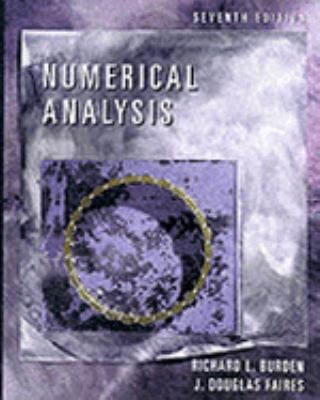130162

9780534382162

# Numerical Analysis\$1.34
\$3.95 Shipping
List Price
\$140.95
Discount
99% Off
You Save
\$139.61

• Condition: Good
• Provider: HippoBooks Contact
• Provider Rating:
85%
• Ships From: Multiple Locations
• Shipping: Standard
• Comments: Spine creases, wear to binding and pages from reading. May contain limited notes, underlining or highlighting that does affect the text. Possible ex library copy, thatll have the markings and stickers associated from the library. Accessories such as CD, codes, toys, may not be included.30-day money back guarantee

Most renters respond to questions in 48 hours or less.
The response will be emailed to you.
• ISBN-13: 9780534382162
• ISBN: 0534382169
• Edition: 7
• Publication Date: 2000
• Publisher: Brooks/Cole

### AUTHOR

Burden, Richard L., Faires, J. Douglas

### SUMMARY

1. MATHEMATICAL PRELIMINARIES Review of Calculus / Round-off Errors and Computer Arithmetic / Algorithms and Convergence / Numerical Software 2. SOLUTIONS OF EQUATIONS IN ONE VARIABLE The Bisection Method / Fixed-Point Iteration / The Newton's Method / Error Analysis for Iterative Methods / Accelerating Convergence / Zeros of Polynomials and Muller's Method / Survey of Methods and Software 3. INTERPOLATION AND POLYNOMIAL APPROXIMATION Interpolation and the LaGrange Polynomial / Divided Differences / Hermite Interpolation / Cubic Spline Interpolation / Parametric Curves / Survey of Methods and Software 4. NUMERICAL DIFFERENTIATION AND INTEGRATION Numerical Differentiation / Richardson's Extrapolation / Elements of Numerical Integration / Composite Numerical Integration / Romberg Integration / Adaptive Quadrature Methods / Gaussian Quadrature / Multiple Integrals / Improper Integrals / Survey of Methods and Software 5. INITIAL-VALUE PROBLEMS FOR ORDINARY DIFFERENTIAL EQUATIONS The Elementary Theory of Initial-Value Problems / Euler's Method / Higher-Order Taylor Methods / Runge-Kutta Methods / Error Control and the Runge-Kutta-Fehlberg Method / Multi-Step Methods / Variable Step-Size Multi-Step Methods / Extrapolation Methods / Higher-Order Equations and Systems of Differential Equations / Stability / Stiff Differential Equations / Survey of Methods and Software 6. DIRECT METHODS FOR SOLVING LINEAR SYSTEMS Linear Systems of Equations / Pivoting Strategies / Linear Algebra and Matrix Inversion / The Determinant of a Matrix / Matrix Factorization / Special Types of Matrices / Survey of Methods and Software 7. ITERATIVE TECHNIQUES IN MATRIX ALGEBRA Norms of Vectors and Matrices / Eigenvalues and Eigenvectors / Iterative Techniques for Solving Linear Systems / Error Bounds and Iterative Refinement / The Conjugate Gradient Method / Survey of Methods and Software 8. APPROXIMATION THEORY Discrete Least Squares Approximation / Orthogonal Polynomials and Least Squares Approximation / Chebyshev Polynomials and Economization of Power Series / Rational Function Approximation / Trigonometric Polynomial Approximation / Fast Fourier Transforms / Survey of Methods and Software 9. APPROXIMATING EIGENVALUES Linear Algebra and Eigenvalues / The Power Method / Householder's Method / The QR Algorithm / Survey of Methods and Software 10. NUMERICAL SOLUTIONS OF NONLINEAR SYSTEMS OF EQUATIONS Fixed Points for Functions of Several Variables / Newton's Method / Quasi-Newton Methods / Steepest Descent Techniques / Homotopy and Continuation Methods / Survey of Methods and Software 11. BOUNDARY-VALUE PROBLEMS FOR ORDINARY DIFFERENTIAL EQUATIONS The Linear Shooting Method / The Shooting Method for Nonlinear Problems / Finite-Difference Methods for Linear Problems / Finite-Difference Methods for Nonlinear Problems / The Rayleigh-Ritz Method / Survey of Methods and Software 12. NUMERICAL SOLUTIONS TO PARTIAL DIFFERENTIAL EQUATIONS Elliptic Partial-Differential Equations / Parabolic Partial-Differential Equations / Hyperbolic Partial-Differential Equations / An Introduction to the Finite-Element Method / Survey of Methods and Software / BIBLIOGRAPHY / ANSWERS TO SELECTED EXERCISES / INDEXBurden, Richard L. is the author of 'Numerical Analysis', published 2000 under ISBN 9780534382162 and ISBN 0534382169.

You can find lots of answers to common customer questions in our FAQs

View a detailed breakdown of our shipping prices